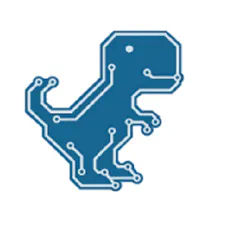# DIY simplest IV9 Numitron clock with Arduino

Nice looking and simple to build Retro style Numitron tubes clock.

IntermediateFull instructions provided2,740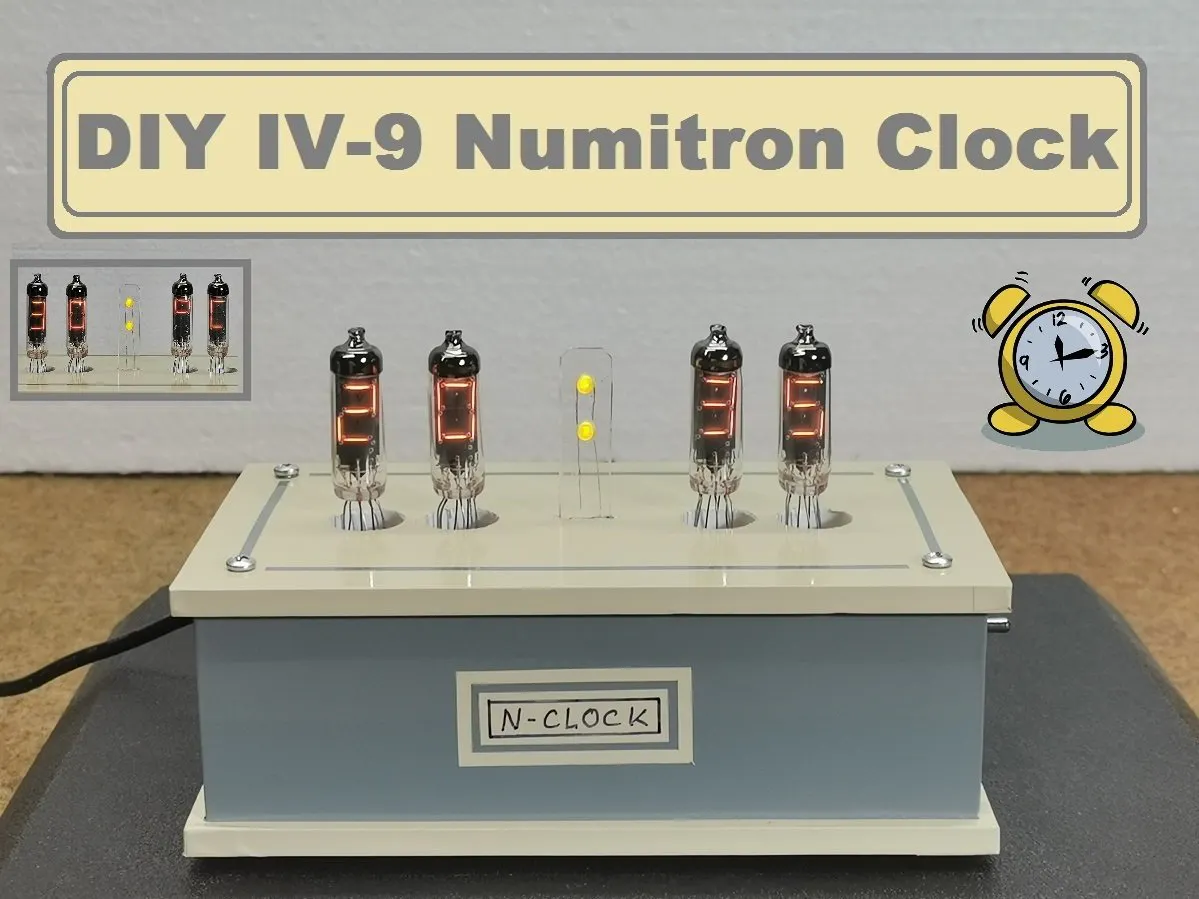## Things used in this project

### Hardware componentsArduino Nano R3
×1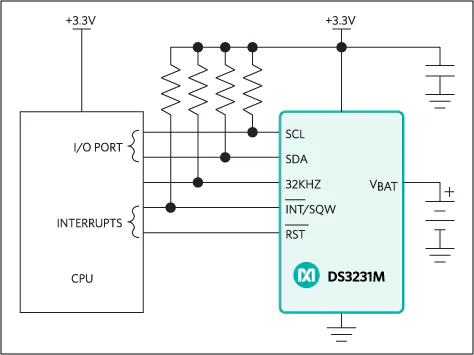Maxim Integrated DS3231M - ±5ppm, I2C Real-Time Clock
×1
 TPIC6C595 shift register
×4
 IV9 Numitron tube
×4LED (generic)
×2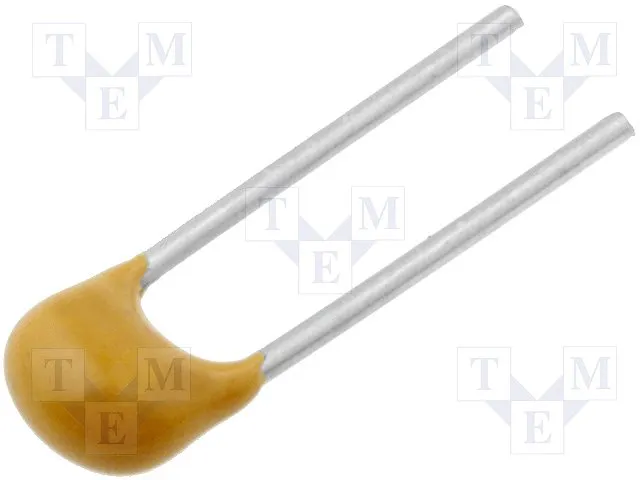Capacitor 100 nF
×4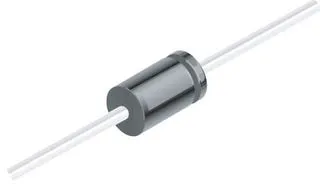1N4007 – High Voltage, High Current Rated Diode
×2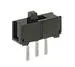Slide Switch
×1Resistor 1k ohm
×1

### Software apps and online servicesArduino IDE

### Hand tools and fabrication machinesSoldering iron (generic)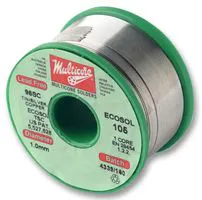## Schematics

### Schematic diagram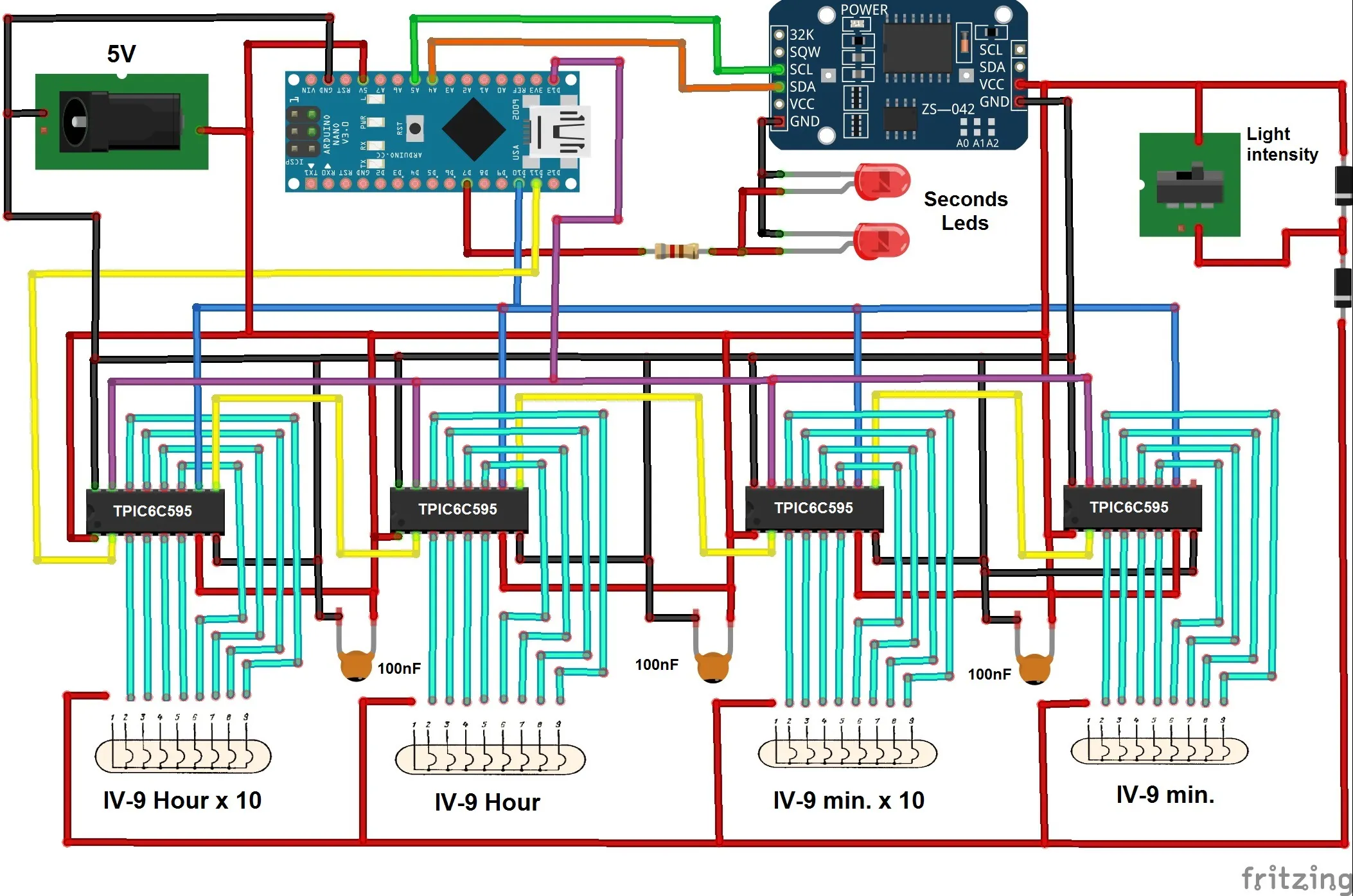## Code

### Arduino Code

C/C++
```#include <RTClib.h> //rtc with temperature for ds3231
RTC_DS3231 rtc; //setting which rtc module is used

#include <Wire.h> //wiring
#include <TimeLib.h> //time lib
#include <Time.h> //time function
#include <DS1307RTC.h> //rtc
#include <SPI.h>
#define led 7
const int latchPin = 13; //latch
const int clockPin = 10; //clock
const int dataPin = 11; //data

unsigned long previousMillis = 0;        // stores last time Led blinked

long interval = 30000;           // interval at which to blink (milliseconds)

unsigned long previousMillisDiode = 0;        // stores last time Led blinked

long intervalDiode = 500;           // interval at which to blink (milliseconds)

const int nums = { //setting display array - according to docs: pin1 is common, pin2 is dot(unused in sketch), rest should be connected to shift registers one by one
0b10111110, //0
0b00000110, //1
0b01111010, //2
0b01101110, //3
0b11000110, //4
0b11101100, //5
0b11111100, //6
0b00001110, //7
0b11111110, //8
0b11101110, //9
0b11001010, //st.
0b10111000 //celz.
};

int hour1; //hour first number
int hour2; //hour second number
int minute1; //minutes first number
int minute2; //minutes second number
int day1; //day first number
int day2; //day second number
int month1; //month first number
int month2; //month second number
int year1; //year first number - constant 2
int year2; //year second number - constant 0 (you wanna live that long to change it?)
int year3; //year third number
int year4; //year fourth number
int hourDecimal; //decimal parsing of hour
int minuteDecimal; //decimal parsing of minute
int dayDecimal; //decimal parsing of day
int monthDecimal; //decimal parsing of month
int year70; //year after unix epoch
int temp1; //first temperature number
int temp2; //second temperature number
int tempDecimal; //decimal parsing of temperature(first two numbers)

void setup() {
pinMode (led,OUTPUT);
pinMode(latchPin, OUTPUT); //set pins to output so you can control the shift register
pinMode(clockPin, OUTPUT); //set pins to output so you can control the shift register
pinMode(dataPin, OUTPUT); //set pins to output so you can control the shift register
Serial.begin(9600);
// initialize SPI:
SPI.begin();
// take the SS pin low to select the chip:
digitalWrite(clockPin,LOW);
}

void loop() {

tmElements_t tm; //naming from DS1307RTC library

minuteDecimal = tm.Minute / 10; //parse output to be readable(shorter) by dividing by ten
hourDecimal = tm.Hour / 10; //parse output to be readable(shorter) by dividing by ten

dayDecimal = tm.Day / 10; //parse output to be readable(shorter) by dividing by ten
monthDecimal = tm.Month / 10; //parse output to be readable(shorter) by dividing by ten

year70 = tm.Year - 30; //display real year by subtracting from unix epoch(1970)

hour1 = hourDecimal; //simple as that
hour2 = tm.Hour - 10 * hourDecimal; //make calculations to display only second number from two digits string
minute1 = minuteDecimal; //simple
minute2 = tm.Minute - 10 * minuteDecimal; //make calculations to display only second number from two digits string

day1 = dayDecimal; //simple
day2 = tm.Day - 10 * dayDecimal; //make calculations to display only second number from two digit string
month1 = monthDecimal; //simple
month2 = tm.Month - 10 * monthDecimal; //make calculations to display only second number from two digit string

year1 = 2; //first year number, do you really need to change that? you got flying cars and etc?
year2 = 0; //second year number, if you need to change that you should be playing with grandkids instead
year3 = year70 / 10; //parse output to be readable(shorter) by dividing by ten
year4 = year70 - 10 * year3; //make calculations to display only second number from two digit string

tempDecimal = rtc.getTemperature()/10; //parse output to be readable(shorter) by dividing by ten

temp1 = tempDecimal; //simple
temp2 = rtc.getTemperature() - 10 * tempDecimal; //make calculations to display only second number from two digit string

if (millis() - previousMillisDiode >= intervalDiode) {
previousMillisDiode = millis();
}

if (millis() - previousMillis >= interval) {
previousMillis = millis();

digitalWrite (clockPin, LOW);
SPI.transfer (0b00000000);
SPI.transfer (0b00000000);
SPI.transfer (0b00000000);
SPI.transfer (0b00000000);
digitalWrite (clockPin, HIGH);

delay(500); //numitron tured off for 0.5 sec to make 'breathing' effect

digitalWrite (clockPin, LOW);
SPI.transfer (nums[month2]);
SPI.transfer (nums[month1]);
SPI.transfer (nums[day2]);
SPI.transfer (nums[day1]);
digitalWrite (clockPin, HIGH);
delay(1500);

digitalWrite (clockPin, LOW);
SPI.transfer (0b00000000);
SPI.transfer (0b00000000);
SPI.transfer (0b00000000);
SPI.transfer (0b00000000);
digitalWrite (clockPin, HIGH);

delay(500); //numitron tured off for 0.5 sec to make 'breathing' effect

digitalWrite (clockPin, LOW);
SPI.transfer (nums[year4]);
SPI.transfer (nums[year3]);
SPI.transfer (nums[year2]);
SPI.transfer (nums[year1]);
digitalWrite (clockPin, HIGH);

delay(1500);

digitalWrite (clockPin, LOW);
SPI.transfer (0b00000000);
SPI.transfer (0b00000000);
SPI.transfer (0b00000000);
SPI.transfer (0b00000000);
digitalWrite (clockPin, HIGH);

delay(500); //numitron tured off for 0.5 sec to make 'breathing' effect

digitalWrite (clockPin, LOW);
SPI.transfer (0b10111000);
SPI.transfer (0b11001010);
SPI.transfer (nums[temp2]);
SPI.transfer (nums[temp1]);
digitalWrite (clockPin, HIGH);

delay(1500);

digitalWrite (clockPin, LOW);
SPI.transfer (0b00000000);
SPI.transfer (0b00000000);
SPI.transfer (0b00000000);
SPI.transfer (0b00000000);
digitalWrite (clockPin, HIGH);

delay(500); //numitron tured off for 0.5 sec to make 'breathing' effect
}
else
{
digitalWrite (clockPin, LOW);
SPI.transfer (nums[minute2]);
SPI.transfer (nums[minute1]);
SPI.transfer (nums[hour2]);
SPI.transfer (nums[hour1]);
digitalWrite (clockPin, HIGH);
}
}
```

## Credits

### Mirko Pavleski

52 projects • 908 followers